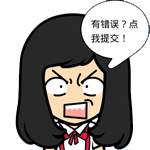# PHPCMS v9调用全站文章

```
/**
* 列表页标签：主要返回的是主表中数据与附表中数据
* @param \$data
*/
public function lists_all(\$data) {
\$this->db->set_model(1);//将模型手动指定为文章模型，为什么？因为根据源代码的套路，模型id的是根据catid来设置的，但是现在获取的是所有的文章，而不局限于某个栏目，所以只能手动将其设置为文章模型1  //根据模型id获取当前模型所对应的模型表和数据表 1：文档模型-news  3：图片模型-picture   2：下载模型-download
if(isset(\$data['where'])) {                                         //如果pc标签中设置了where属性，一般情况下不存在
\$sql = \$data['where'];
} else {                                                            //如果pc标签中没有设置where属性
\$thumb = intval(\$data['thumb']) ? " AND thumb != ''" : '';      //如果有thumb属性
\$sql = "status=99 ".\$thumb;//去掉catid条件              //如果当前栏目下不存在子栏目
}
\$order = \$data['order'];                                            //pc标签中order属性
//\$sql作为一个条件出现，调用的是model.calss.php文件中的select方法，返回结果集数组，并按照键名'id'排序
\$return = \$this->db->select(\$sql, '*', \$data['limit'], \$order, '', 'id');//从数据库中获取主表数据，使用的也是sql语句查询

//如果需要在前台显示类别名称，可添加如下代码

\$TYPES = getcache('type_content','commons');//获取类别缓存文件，此文件缓存了所有与类别信息相关的信息
foreach (\$return as \$key=>\$v) {
\$return[\$key][typename]=\$TYPES[\$v['typeid']][name];//给\$return中的每篇文章追加一个类别字段
}
//echo "";
//print_r(\$return);

//调用副表的数据
if (isset(\$data['moreinfo']) && intval(\$data['moreinfo']) == 1) {   //如果pc标签中设置了moreinfo属性：是否调用附表数据
\$ids = array();
//\$return为返回的主表数据
foreach (\$return as \$v) {                                       //循环主表中的记录信息:\$v-主表中的每条记录
if (isset(\$v['id']) && !empty(\$v['id'])) {
\$ids[] = \$v['id'];                                      //ids[]是主表中排序完成的文章id数组
} else {
continue;
}
}
if (!empty(\$ids)) {
\$this->db->table_name = \$this->db->table_name.'_data';      //副表名
\$ids = implode('\',\'', \$ids);                              //以逗号拼接成一个字符串
\$r = \$this->db->select("`id` IN ('\$ids')", '*', '', '', '', 'id');//查询与主表中数据有关的副表中的数据
if (!empty(\$r)) {
foreach (\$r as \$k=>\$v) {                             //副表中的数据
//\$return:返回的主表中的数据，主表中的id字段值与附表中的id字段值是对应的，也就是说一篇文章在主表中存储的id跟在附表中存储的id的值是相等的
if (isset(\$return[\$k])) \$return[\$k] = array_merge(\$v, \$return[\$k]);//主表中数据与副表中数据合并
}
}
}
}
return \$return;
}

调用标签：
{pc:content action="lists_all" num="15" order="id DESC" page="\$page"}
{loop \$data \$r \$val}{\$val[title]}
{str_cut(\$val[description],220,'...')}
{date('Y年m月d日 H:i:s',\$val[inputtime])}     {date('H:i',\$val[updatetime])}更新

{/loop}
{/pc}

```